# Class and Constructor in C++

## Class and Constructor in C++

In this article, I am going to discuss Class and Constructor in C++ with Examples. Please read our previous article where we discussed how to convert a C Program to C++ Program.

##### Class and Constructor in C++

Let us write a C++ Program to understand the class and constructor in detail. Please have a look at the following to understand class and constructor.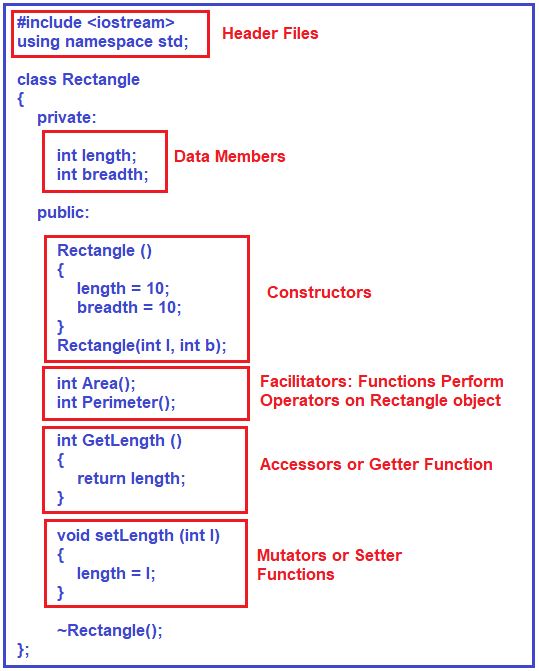First of all, we should include a header file i.e. iostream (it maybe iostream.h depending on the compiler you are using). Then you need to write using namespace std; so, that you can use the ‘cin’ and ‘cout’ objects for display or reading in your program.

##### Class:

Then we write a class called Rectangle using the class keyword.

##### Data Members:

Inside the class Rectangle, we declare two private data members i.e. length and breadth. We are taking both of the data members as integers but if you want to change the data type then you can change that. These two data members are going to hold the length and breadth values of the Rectangle

Note: The next thing is to define the member functions and Constructors. We define all member functions and constructors inside the public block.

##### Constructors:

First, we define a constructor inside the public block. The point that you need to remember is the constructor should not have any return type but it may take or may not take any parameter. Here, first, we are creating a no-argument constructor that doesn’t take any argument. And within the no-argument constructor, we initialize the length and breadth member to 10 and 20 respectively and we can call it the default constructor.

Then, we define another constructor with arguments which are also called a parameterized constructor. This parameterized constructor takes length and breadth parameters. We will be defining this constructor outside using the scope resolution operator. This is just a prototype or a signature of a function or the header of a function.

Note: When we define more than one constructor in a single class, then it is also called as Constructor overloading.

##### Member Functions:

On the rectangle object, we need some functions like calculating the area or calculating the perimeter. So, we define one function called Area with return type as integer and this function is going to return the Area of the Rectangle. We also define the Perimeter function with return type integer which will return the perimeter of the rectangle.

##### Getter Function:

Here I am adding one more function i.e. GetLength which will return the length of the Rectangle and here only we have expanded the function and return the length. This function is also called an Accessor function or Getter Function.

##### Setter Function

Now we need one more function to set the length of the Rectangle and for this purpose, we define the setLength function which will set the length of the Rectangle. Here only we have expanded the function which will set the new length of the Rectangle. This function is also called a Mutator function or Setter Function.

##### Destructor:

If you have any dynamic memory inside the class then you can release those memories using a destructor. A destructor is also the same as the class name but it is preceded by the ‘~’ symbol and no arguments at all.

Now let us implemented the three functions i.e. Rectangle, Area, and Perimeter outside the class definition.

##### Parameterized Constructor Implementation:

For the implementation, first, we need to write the class name Rectangle, then the scope resolution operator i.e. (::) and followed by the function or constructor name with the required parameters as shown in the below image. The only thing that we have to do inside this constructor is length=l; and breadth=b; If at all you have any validations, like whether the length and breadth are valid or not, you can check here.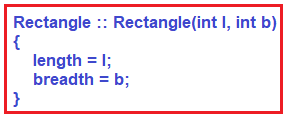##### Area Function:

Let us see how to define the Area function. In order to define it, first, we need to write the return type i.e. int, and then the class name i.e. Rectangle, and then the scope resolution operator (::) followed by the function name i.e. Area as shown in the below image. This function does not take any parameter and returns the area by multiplying the length and breadth.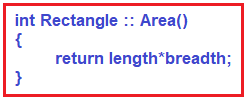##### Perimeter Function:

Let us see how to define the Perimeter function. In order to define it, first, we need to write the return type i.e. int, and then the class name i.e. Rectangle, and then the scope resolution operator (::) followed by the function name i.e. Perimeter as shown in the below image. This function also does not take any parameter and returns the perimeter by adding the length and breadth value and then multiplying the result with 2.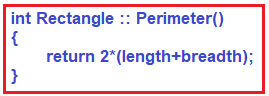##### Destructor:

If you have anything to destroy like if you have any dynamic memory allocation, then you can release those memories using the destructor function. Here, we don’t have to destroy anything so, we are just implementing the destructor function with an empty body. The destructor function does not return any value so no return type. First, write the class name and then the scope resolution operator followed by the destructor function as shown below.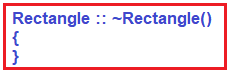These are the functions we have implemented them using the scope resolution operator outside the class. Now let us see how to use all the above functions inside the main function.

##### Main function:

Inside the main function, first, create an object of class Rectangle and while creating the object we are passing the values 10 and 5. Then we call the Area and Perimeter functions on the rectangle object using the dot (.) operator. Then we change the length of the rectangle object to 20 by calling the SetLength function on the rectangle object. And then we display the updated length of the rectangle object by calling the GetLength function as shown in the below image.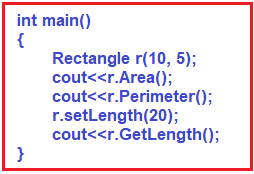Once, the main function ends, the destructor function will be automatically called because the object is going outside the scope.

##### The complete code is given below.
```#include <iostream>
using namespace std;

class Rectangle
{
private:
int length;

public:
Rectangle ()
{
length = 10;
}
Rectangle (int l, int b);

int Area ();
int Perimeter ();

int GetLength ()
{
return length;
}

void setLength (int l)
{
length = l;
}

~Rectangle ();
};

Rectangle::Rectangle (int l, int b)
{
length = l;
}

int Rectangle::Area ()
{
}

int Rectangle::Perimeter ()
{
return 2 * (length + breadth);
}

Rectangle::~Rectangle ()
{
}

int main ()
{
Rectangle r (10, 5);
cout << r.Area ();
cout << r.Perimeter ();
r.setLength (20);
cout << r.GetLength ();
}
```

In the next article, I am going to discuss the Template Classes in C++. Here in this article, I try to explain Class and Constructors in C++. I hope you enjoy this Class and Constructors in the C++ article.